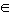SEARCH HOMEMath Central Quandaries & QueriesQuestion from dan, a student: A number X is converted to base 7 and becomes a four digit number. Its leftmost digit is removed and written again as the rightmost digit. The number thus obtained is twice X. Find the decimal representations of all such numbers X.Hi Dan,

[For this reply, I will use red to indicate base 7 numbers and black for decimal numbers. For example, 6027 = 29610.]

Suppose that in base 7, X = abcd7 that is X = 73 a + 72 b + 7 c + d. Since we are working in base 7, the possible values of a, b, c and d are 0, 1, 2, 3, 4, 5 or 6. You know that bcda7 is twice X so

bcda7 = (2)(abcd7)

73 b + 72 c + 7 d + a = 2(73 a + 72 b + 7 c + d)

which can be rewritten

73 (b - 2a) + 72 (c - 2b) + 7 (d - 2c) = 2d - a

The left side of this equation is divisible by 7 so the right side must be divisible by 7. So what combinations of d and a make the right side divisible by 7?

For each of the possible values of d, d = 0, 1, ... 6 I looked for corresponding values of a, if any. I found three possible pairings:

(d, a){ (4, 1), (5, 3), (6, 5) }

The pair (6, 5) doesn't work since a = 5 makes X = 5bcd7 and 2X is a five digit number since (2)(57) = 137.

The pair (5, 3) doesn't work either. Can you see why?

Thus X = 1bc47 and (2)(1bc47) = bc417.

Now perform the multiplication (2)(1bc47). 2 times 4 is 810 which is 117 so there is a carry of 1. Hence for the next digit 2c plus the carry of 1 gives ?47 where ? is some digit, ie (2c + 1)7 = (?4)7 . I then tried all the possibilities for c, c = 0, 1, ..., 6 and found that c = 5. Thus

(2)(1b547) = b5417

Finally perform the multiplication (2)(1b547) and see what value(s) of b give (2)(1b547) = b5417.

PennyMath Central is supported by the University of Regina and The Pacific Institute for the Mathematical Sciences.